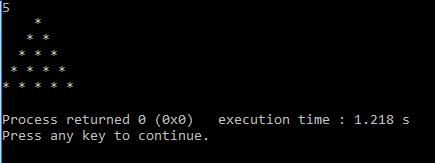# Full Pyramid

``````a=int(input())
for i in range(1,a+1):
for j in range(a-i):
print(end = " ")
for star in range(i):
print("*", end =" ")
print()
``````

Where is my problem?

@corrupted_brain, at least try to read previous posts before posting your problem. You are printing an extra space at the end of every line, fix it and it will do.

Thank you brother , now it is working

Can you please tell me where is the problem in my code:

``````    #include <iostream>
using namespace std;
int main()
{
int i, n, x;
cin >> n;
for(i=1; i<=n; i++){
for(x=0;x<(n-i);x++){
cout << " ";
}
for(x=i;x>0;x--){
cout << "*" << " ";
}
cout << "\n";
}
``````

return 0;
}

It says wrong. but i compiled it in codeblocks:Extra space at the end of every line.
You should read previous posts before you post @Aiden_Pirace.
Cause people tend to do the same mistakes.

1 Like

Where am I putting up the extra space? I have tried a lot, at least all the patterns for this problem, but nothing seems to work in toph,

``````#include<stdio.h>
int main()
{
int n;
scanf("%d",&n);
int r=n;
for(int i=1; i<=r;i++){
for(int j=1; j<=n;j++){
printf(" ");
}
n--;
for(int k=1; k<=i;k++){
printf("* ");
}
printf("\n");
}
return 0;
}
``````

@iker_13in front as well as the end of every line.``````#include<stdio.h>
int main()
{
int a,i,j,k,l;
scanf("%d",&a);
k=a-1;
l=k;

for(i=0;i<a;i++,k--,l--){
for(;k>0;k--){
printf(" ");
}

for(j=0;j<=i;j++){

printf("* ");
}
printf("\n");
k=l;
}
return 0;
}
``````

i got solution

``````[REDACTED]
``````
``````#include<stdio.h>
int main()
{
int i, j, k, n;
scanf("%d", &n);
for (i = 0; i < n; i++)
{
for (j = 0; j < n - i; j++)
printf(" ");
for (k = 0; k <= i; k++)
{
printf("*");
if (k!=i)
printf(" ");
}
if (i!=n-1)
printf("\n");
}
return 0;
}
``````

``````#include<iostream>
using namespace std;
int main()
{
int r,i,j;
cin>>r;
for(i=1;i<=r;i++){
for(j=1;j<=r-i;j++){
cout<<" ";
}
for(j=1;j<=i;j++){
cout<<"* ";
}
cout<<endl;
}
return 0;

}
``````

what is the problem in this code

``````#include <stdio.h>

int main() {
int col,row,N;
scanf("%d",&N);
for(row=1;row<=N;row++)
{
for(col=1;col<=N-row;col++)
printf(" ");
for(col=1;col<=row;col++)
printf("* ");
printf("\n");
}

return 0;
}
``````

Whats the problem ? my output and expected are same

nice problem, learnt something new

In general, building a pyramid by code is not very easy. But I made it by writing only three line codes😁. But I do not understand why my code is not acceptable. What is the problem in my code?.However why my stars are not showing up(one asterisk after print(" "* and one inside of )+"*")

Python 3

``````n = int(input())
for i in range(n):
print(" "*(n-(1+i))+"* "*(1+i))
``````
``````   Scanner input=new Scanner(System.in);
int N;
N=input.nextInt();
for (int r =1; r<=N; r++)
{
for (int c = 1; c <=N-r; c++){
System.out.print(" ");
}
for (int c =1; c <=r; c++) {
System.out.print(" *");
}

System.out.println();
}
``````

wthats the problem in my code ?

It seems that you have solved it yourself.

#include <stdio.h>
int main()
{
int n,row,col;
scanf("%d",&n);
for(row=1;row<=n;row++){
for(col=1;col<=n-row;col++)
printf(" “);
for(col=1;col<=row;col++)
printf(”* ");

``````        printf("\n");
}
return 0;
``````

}

/What’s the problem in my code…anyone plz find the bug.Each time I run this it shows WA in the first case/

``````the program...
package hello;
import java.util.Scanner;

public class myp {

public static void main(String[] args) {

//System.out.println("Write somthing:");

Scanner x =new Scanner(System.in);

int a=x.nextInt();

for(int i=0;i<a;i++) {
for(int d=i;d<a-1;d++) {
System.out.print(" ");
}
for(int j=0;j<=i;j++) {

System.out.print("* ");
}

System.out.print("\n");
}
}

}
``````

#Could anyone tell me the problem of my code:
N=int(input())
for i in range(N):
print(’{}{}’.format(" “*(N-(i+1)),” "(i+1)))

What’s the problem of this code?!

n=int(input())
c=1
while(c<=n):
s=""